# class TSpectrum3: public TNamed

```THIS CLASS CONTAINS ADVANCED SPECTRA PROCESSING FUNCTIONS.

THREE-DIMENSIONAL BACKGROUND ESTIMATION FUNCTIONS
THREE-DIMENSIONAL SMOOTHING FUNCTIONS
THREE-DIMENSIONAL DECONVOLUTION FUNCTIONS
THREE-DIMENSIONAL PEAK SEARCH FUNCTIONS

These functions were written by:
Miroslav Morhac
Institute of Physics
Dubravska cesta 9, 842 28 BRATISLAVA
SLOVAKIA

email:fyzimiro@savba.sk,    fax:+421 7 54772479

The original code in C has been repackaged as a C++ class by R.Brun

The algorithms in this class have been published in the following
references:
  M.Morhac et al.: Background elimination methods for
multidimensional coincidence gamma-ray spectra. Nuclear
Instruments and Methods in Physics Research A 401 (1997) 113-
132.

  M.Morhac et al.: Efficient one- and two-dimensional Gold
deconvolution and its application to gamma-ray spectra
decomposition. Nuclear Instruments and Methods in Physics
Research A 401 (1997) 385-408.

 M. Morhac et al.: Efficient algorithm of multidimensional
deconvolution and its application to nuclear data processing. Digital
Signal Processing, Vol. 13, No. 1, (2003), 144-171.

  M.Morhac et al.: Identification of peaks in multidimensional
coincidence gamma-ray spectra. Nuclear Instruments and Methods in
Research Physics A  443(2000), 108-125.

These NIM papers are also available as Postscript files from:

ftp://root.cern.ch/root/SpectrumDec.ps.gz
ftp://root.cern.ch/root/SpectrumSrc.ps.gz
ftp://root.cern.ch/root/SpectrumBck.ps.gz

```

## Function Members (Methods)

public:
protected:
 virtual void TObject::DoError(int level, const char* location, const char* fmt, va_list va) const void TObject::MakeZombie()

## Data Members

public:
 enum { kBackIncreasingWindow kBackDecreasingWindow kBackSuccessiveFiltering kBackOneStepFiltering }; enum TObject::EStatusBits { kCanDelete kMustCleanup kObjInCanvas kIsReferenced kHasUUID kCannotPick kNoContextMenu kInvalidObject }; enum TObject::[unnamed] { kIsOnHeap kNotDeleted kZombie kBitMask kSingleKey kOverwrite kWriteDelete };
protected:
 TH1* fHistogram resulting histogram Int_t fMaxPeaks Maximum number of peaks to be found Int_t fNPeaks number of peaks found TString TNamed::fName object identifier Float_t* fPosition [fNPeaks] array of current peak positions Float_t* fPositionX [fNPeaks] X positions of peaks Float_t* fPositionY [fNPeaks] Y positions of peaks Float_t* fPositionZ [fNPeaks] Z positions of peaks Float_t fResolution resolution of the neighboring peaks TString TNamed::fTitle object title

## Class Charts## Function documentation

``` Constructor.
```
TSpectrum3(Int_t maxpositions, Float_t resolution = 1)
```  maxpositions:  maximum number of peaks
resolution:    determines resolution of the neighboring peaks
default value is 1 correspond to 3 sigma distance
between peaks. Higher values allow higher resolution
(smaller distance between peaks.
May be set later through SetResolution.
```

``` Destructor.
```
const char * Background(const TH1* hist, int niter, Option_t* option = "goff")
```ONE-DIMENSIONAL BACKGROUND ESTIMATION FUNCTION
This function calculates background spectrum from source in h.
The result is placed in the vector pointed by spectrum pointer.

Function parameters:
spectrum:  pointer to the vector of source spectrum
size:      length of spectrum and working space vectors
number_of_iterations, for details we refer to manual

```
void Print(Option_t* option = "") const
``` Print the array of positions
```
Int_t Search(const TH1* hist, Double_t sigma = 2, Option_t* option = "goff", Double_t threshold = 0.05)
```ONE-DIMENSIONAL PEAK SEARCH FUNCTION
This function searches for peaks in source spectrum in hin
The number of found peaks and their positions are written into
the members fNpeaks and fPositionX.

Function parameters:
hin:       pointer to the histogram of source spectrum
sigma:   sigma of searched peaks, for details we refer to manual
Note that sigma is in number of bins
threshold: (default=0.05)  peaks with amplitude less than

if option is not equal to "goff" (goff is the default), then
a polymarker object is created and added to the list of functions of
the histogram. The histogram is drawn with the specified option and
the polymarker object drawn on top of the histogram.
The polymarker coordinates correspond to the npeaks peaks found in
the histogram.
A pointer to the polymarker object can be retrieved later via:
TList *functions = hin->GetListOfFunctions();
TPolyMarker *pm = (TPolyMarker*)functions->FindObject("TPolyMarker")

```
void SetResolution(Float_t resolution = 1)
```  resolution: determines resolution of the neighboring peaks
default value is 1 correspond to 3 sigma distance
between peaks. Higher values allow higher resolution
(smaller distance between peaks.
May be set later through SetResolution.
```
const char * Background(float*** spectrum, Int_t ssizex, Int_t ssizey, Int_t ssizez, Int_t numberIterationsX, Int_t numberIterationsY, Int_t numberIterationsZ, Int_t direction, Int_t filterType)
```THREE-DIMENSIONAL BACKGROUND ESTIMATION FUNCTIONS
This function calculates background spectrum from source spectrum.
The result is placed to the array pointed by spectrum pointer.

Function parameters:
spectrum-pointer to the array of source spectrum
ssizex-x length of spectrum
ssizey-y length of spectrum
ssizez-z length of spectrum
numberIterationsX-maximal x width of clipping window
numberIterationsY-maximal y width of clipping window
numberIterationsZ-maximal z width of clipping window
for details we refer to manual
direction- direction of change of clipping window
- possible values=kBackIncreasingWindow
kBackDecreasingWindow
filterType-determines the algorithm of the filtering
-possible values=kBackSuccessiveFiltering
kBackOneStepFiltering

```

Background estimation

Goal: Separation of useful information (peaks) from useless information (background)

         method is based on Sensitive Nonlinear Iterative Peak (SNIP) clipping algorithm 

         there exist two algorithms for the estimation of new value in the channel 

Algorithm based on Successive Comparisons

It is an extension of one-dimensional SNIP algorithm to another dimension. For details we refer to .

Algorithm based on One Step Filtering

The algorithm is analogous to that for 2-dimensional data. For details we refer to TSpectrum2. New value in the estimated channel is calculated aswhere p = 1, 2, , number_of_iterations.

Function:

const char* TSpectrum3::Background (float ***fSpectrum, int fSizex, int fSizey, int fSizez, int fNumberIterationsX, int fNumberIterationsY, int fNumberIterationsZ,  int fDirection, int fFilterType)

This function calculates background spectrum from the source spectrum.  The result is placed in the matrix pointed by fSpectrum pointer.  One can also switch the direction of the change of the clipping window and to select one of the two above given algorithms. On successful completion it returns 0. On error it returns pointer to the string describing error.

Parameters:

fSpectrum-pointer to the matrix of source spectrum

fSizex, fSizey, fSizez -lengths of the spectrum matrix

fNumberIterationsX, fNumberIterationsY, fNumberIterationsZ maximal

widths of clipping window,

fDirection- direction of change of clipping window

- possible values=kBackIncreasingWindow

kBackDecreasingWindow

fFilterType-type of the clipping algorithm,

-possible values=kBack SuccessiveFiltering

kBackOneStepFiltering

References:

  C. G Ryan et al.: SNIP, a statistics-sensitive background treatment for the quantitative analysis of PIXE spectra in geoscience applications. NIM, B34 (1988), 396-402.

  M. Morháč, J. Kliman, V. Matouek, M. Veselský, I. Turzo.: Background elimination methods for multidimensional gamma-ray spectra. NIM, A401 (1997) 113-132.

Example 1 script Back3.c :Fig. 1 Original three-dimensional gamma-gamma-gamma-ray spectrumFig. 2 Background estimated from data from Fig. 1 using decreasing clipping window with widths 5, 5, 5 and algorithm based on successive comparisons. The estimate includes not only continuously changing background but also one- and two-dimensional ridges.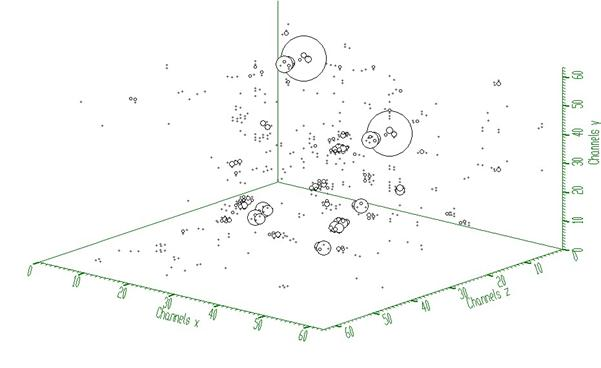Fig. 3 Resulting peaks after subtraction of the estimated background (Fig. 2) from original three-dimensional gamma-gamma-gamma-ray spectrum (Fig. 1).

Script:

// Example to illustrate the background estimator (class TSpectrum3).

// To execute this example, do

// root > .x Back3.C

void Back3() {

Int_t i, j, k;

Int_t nbinsx = 64;

Int_t nbinsy = 64;

Int_t nbinsz = 64;

Int_t xmin  = 0;

Int_t xmax  = nbinsx;

Int_t ymin  = 0;

Int_t ymax  = nbinsy;

Int_t zmin  = 0;

Int_t zmax  = nbinsz;

float *** source = new float **[nbinsx];

float *** dest = new float **[nbinsx];

for(i=0;i<nbinsx;i++){

source[i]=new float* [nbinsy];

for(j=0;j<nbinsy;j++)

source[i][j]=new float [nbinsz];

}

for(i=0;i<nbinsx;i++){

dest[i]=new float* [nbinsy];

for(j=0;j<nbinsy;j++)

dest[i][j]=new float [nbinsz];

}

TH3F *back = new TH3F("back","Background estimation",nbinsx,xmin,xmax,nbinsy,ymin,ymax,nbinsz,zmin,zmax);

TFile *f = new TFile("TSpectrum3.root");

back=(TH3F*) f->Get("back;1");

TCanvas *Background = new TCanvas("Background","Estimation of background with decreasing window",10,10,1000,700);

TSpectrum3 *s = new TSpectrum3();

for (i = 0; i < nbinsx; i++){

for (j = 0; j < nbinsy; j++){

for (k = 0; k < nbinsz; k++){

source[i][j][k] = back->GetBinContent(i + 1,j + 1,k + 1);

dest[i][j][k] = back->GetBinContent(i + 1,j + 1,k + 1);

}

}

}

s->Background(dest,nbinsx,nbinsy,nbinsz,5,5,5,s->kBackDecreasingWindow,s->kBackSuccessiveFiltering);

for (i = 0; i < nbinsx; i++){

for (j = 0; j < nbinsy; j++){

for (k = 0; k < nbinsz; k++){

back->SetBinContent(i + 1,j + 1,k + 1, dest[i][j][k]);

}

}

}

FILE *out;

char PATH;

strcpy(PATH,"spectra3\\back_output_5ds.spe");

out=fopen(PATH,"wb");

for(i=0;i<nbinsx;i++){

for(j=0;j<nbinsy;j++){

fwrite(dest[i][j], sizeof(dest),nbinsz,out);

}

}

fclose(out);

for (i = 0; i < nbinsx; i++){

for (j = 0; j < nbinsy; j++){

for (k = 0; k < nbinsz; k++){

source[i][j][k] = source[i][j][k] - dest[i][j][k];

}

}

}

for (i = 0; i < nbinsx; i++){

for (j = 0; j < nbinsy; j++){

for (k = 0; k < nbinsz; k++){

back->SetBinContent(i + 1,j + 1,k + 1, source[i][j][k]);

}

}

}

strcpy(PATH,"spectra3\\back_peaks_5ds.spe");

out=fopen(PATH,"wb");

for(i=0;i<nbinsx;i++){

for(j=0;j<nbinsy;j++){

fwrite(source[i][j], sizeof(source),nbinsz,out);

}

}

fclose(out);

back->Draw("");

}

const char* SmoothMarkov(float*** source, Int_t ssizex, Int_t ssizey, Int_t ssizez, Int_t averWindow)
```THREE-DIMENSIONAL MARKOV SPECTRUM SMOOTHING FUNCTION

This function calculates smoothed spectrum from source spectrum
based on Markov chain method.
The result is placed in the array pointed by spectrum pointer.

Function parameters:
source-pointer to the array of source spectrum
working_space-pointer to the working array
ssizex-x length of spectrum and working space arrays
ssizey-y length of spectrum and working space arrays
ssizey-z length of spectrum and working space arrays
averWindow-width of averaging smoothing window

```

Smoothing

Goal: Suppression of statistical fluctuations

         the algorithm is based on discrete Markov chain, which has very simple invariant distributionbeing defined from the normalization conditionn is the length of the smoothed spectrum andis the probability of the change of the peak position from channel i to the channel i+1.is the normalization constant so thatand m is a width of smoothing window. We have extended this algorithm to three dimensions.

Function:

const char* TSpectrum3::SmoothMarkov(float ***fSpectrum, int fSizex, int fSizey, int fSizey,  int fAverWindow)

This function calculates smoothed spectrum from the source spectrum based on Markov chain method. The result is placed in the field pointed by source pointer. On successful completion it returns 0. On error it returns pointer to the string describing error.

Parameters:

fSpectrum-pointer to the matrix of source spectrum

fSizex, fSizey, fSizez -lengths of the spectrum matrix

fAverWindow-width of averaging smoothing window

Reference:

 Z.K. Silagadze, A new algorithm for automatic photopeak searches. NIM A 376 (1996), 451.

Example 1  script SmootMarkov3.c :Fig. 1 Original noisy spectrum.Fig. 2 Smoothed spectrum with averaging window m=3.

Script:

// Example to illustrate the Markov smoothing (class TSpectrum3).

// To execute this example, do

// root > .x SmoothMarkov3.C

void SmoothMarkov3() {

Int_t i, j, k;

Int_t nbinsx = 64;

Int_t nbinsy = 64;

Int_t nbinsz = 64;

Int_t xmin  = 0;

Int_t xmax  = nbinsx;

Int_t ymin  = 0;

Int_t ymax  = nbinsy;

Int_t zmin  = 0;

Int_t zmax  = nbinsz;

float *** source = new float **[nbinsx];

for(i=0;i<nbinsx;i++){

source[i]=new float* [nbinsy];

for(j=0;j<nbinsy;j++)

source[i][j]=new float [nbinsz];

}

TH3F *sm = new TH3F("Smoothing","Markov smoothing",nbinsx,xmin,xmax,nbinsy,ymin,ymax,nbinsz,zmin,zmax);

TFile *f = new TFile("TSpectrum3.root");

sm=(TH3F*) f->Get("back;1");

TCanvas *Background = new TCanvas("Smoothing","Markov smoothing",10,10,1000,700);

TSpectrum3 *s = new TSpectrum3();

for (i = 0; i < nbinsx; i++){

for (j = 0; j < nbinsy; j++){

for (k = 0; k < nbinsz; k++){

source[i][j][k] = sm->GetBinContent(i + 1,j + 1,k + 1);

}

}

}

s->SmoothMarkov(source,nbinsx,nbinsy,nbinsz,3);

for (i = 0; i < nbinsx; i++){

for (j = 0; j < nbinsy; j++){

for (k = 0; k < nbinsz; k++){

sm->SetBinContent(i + 1,j + 1,k + 1, source[i][j][k]);

}

}

}

sm->Draw("");

}

const char * Deconvolution(float*** source, const float*** resp, Int_t ssizex, Int_t ssizey, Int_t ssizez, Int_t numberIterations, Int_t numberRepetitions, Double_t boost)
```THREE-DIMENSIONAL DECONVOLUTION FUNCTION
This function calculates deconvolution from source spectrum
according to response spectrum
The result is placed in the cube pointed by source pointer.

Function parameters:
source-pointer to the cube of source spectrum
resp-pointer to the cube of response spectrum
ssizex-x length of source and response spectra
ssizey-y length of source and response spectra
ssizey-y length of source and response spectra
numberIterations, for details we refer to manual
numberRepetitions, for details we refer to manual
boost, boosting factor, for details we refer to manual

```

Deconvolution

Goal: Improvement of the resolution in spectra, decomposition of multiplets

Mathematical formulation of the 3-dimensional convolution system is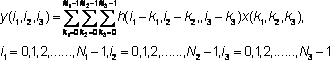where h(i,j,k) is the impulse response function, x, y are input and output fields, respectively,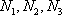, are the lengths of x and h fields

         let us assume that we know the response and the output fields (spectra) of the above given system.

         the deconvolution represents solution of the overdetermined system of linear equations, i.e.,  the calculation of the field x.

         from numerical stability point of view the operation of deconvolution is extremely critical (ill-posed  problem) as well as time consuming operation.

         the Gold deconvolution algorithm proves to work very well even for 2-dimensional systems. Generalization of the algorithm for 2-dimensional systems was presented in , and for multidimensional systems in .

         for Gold deconvolution algorithm as well as for boosted deconvolution algorithm we refer also to TSpectrum and TSpectrum2

Function:

const char* TSpectrum3::Deconvolution(float ***fSource, const float ***fResp, int fSizex, int fSizey, int fSizez, int fNumberIterations, int fNumberRepetitions, double fBoost)

This function calculates deconvolution from source spectrum according to response spectrum using Gold deconvolution algorithm. The result is placed in the field pointed by source pointer. On successful completion it returns 0. On error it returns pointer to the string describing error. If desired after every fNumberIterations one can apply boosting operation (exponential function with exponent given by fBoost coefficient) and repeat it fNumberRepetitions times.

Parameters:

fSource-pointer to the matrix of source spectrum

fResp-pointer to the matrix of response spectrum

fSizex, fSizey, fSizez -lengths of the spectrum matrix

fNumberIterations-number of iterations

fNumberRepetitions-number of repetitions for boosted deconvolution. It must be

greater or equal to one.

fBoost-boosting coefficient, applies only if fNumberRepetitions is greater than one.

Recommended range <1,2>.

References:

 M. Morháč, J. Kliman, V. Matouek, M. Veselský, I. Turzo.: Efficient one- and two-dimensional Gold deconvolution and its application to gamma-ray spectra decomposition. NIM, A401 (1997) 385-408.

 Morháč M., Matouek V., Kliman J., Efficient algorithm of multidimensional deconvolution and its application to nuclear data processing, Digital Signal Processing 13 (2003) 144.

Example 1  script Decon.c :

         response function (usually peak) should be shifted to the beginning of the coordinate system (see Fig. 1)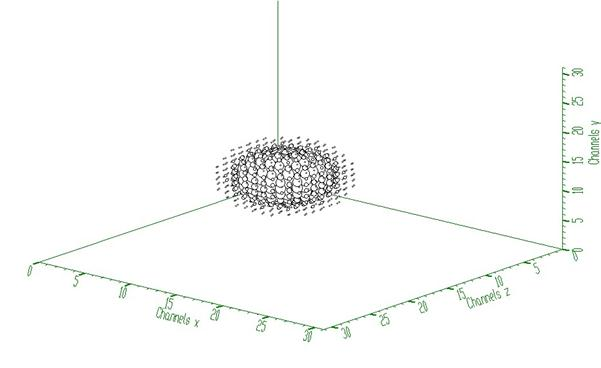Fig. 1 Three-dimensional response spectrum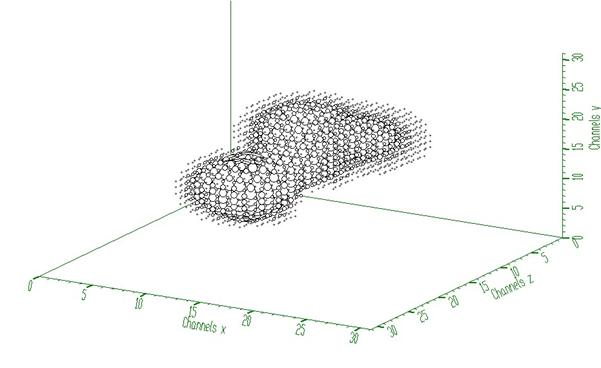Fig. 2 Three-dimensional input spectrum (before deconvolution)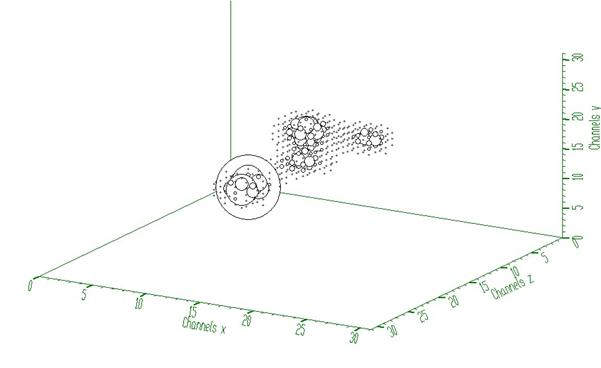Fig. 3 Spectrum from Fig. 2 after deconvolution (100 iterations)

Script:

// Example to illustrate the Gold deconvolution (class TSpectrum3).

// To execute this example, do

// root > .x Decon3.C

#include <TSpectrum3>

void Decon3() {

Int_t i, j, k;

Int_t nbinsx = 32;

Int_t nbinsy = 32;

Int_t nbinsz = 32;

Int_t xmin  = 0;

Int_t xmax  = nbinsx;

Int_t ymin  = 0;

Int_t ymax  = nbinsy;

Int_t zmin  = 0;

Int_t zmax  = nbinsz;

float *** source = new float **[nbinsx];

float *** resp = new float **[nbinsx];

for(i=0;i<nbinsx;i++){

source[i]=new float* [nbinsy];

for(j=0;j<nbinsy;j++)

source[i][j]=new float [nbinsz];

}

for(i=0;i<nbinsx;i++){

resp[i]=new float* [nbinsy];

for(j=0;j<nbinsy;j++)

resp[i][j]=new float [nbinsz];

}

TH3F *decon_in = new TH3F("decon_in","Deconvolution",nbinsx,xmin,xmax,nbinsy,ymin,ymax,nbinsz,zmin,zmax);

TH3F *decon_resp = new TH3F("decon_resp","Deconvolution",nbinsx,xmin,xmax,nbinsy,ymin,ymax,nbinsz,zmin,zmax);

TFile *f = new TFile("TSpectrum3.root");

decon_in=(TH3F*) f->Get("decon_in;1");

decon_resp=(TH3F*) f->Get("decon_resp;1");

TCanvas *Deconvolution = new TCanvas("Deconvolution","Deconvolution of 3-dimensional spectra",10,10,1000,700);

TSpectrum3 *s = new TSpectrum3();

for (i = 0; i < nbinsx; i++){

for (j = 0; j < nbinsy; j++){

for (k = 0; k < nbinsz; k++){

source[i][j][k] = decon_in->GetBinContent(i + 1,j + 1,k + 1);

resp[i][j][k] = decon_resp->GetBinContent(i + 1,j + 1,k + 1);

}

}

}

s->Deconvolution(source,resp,nbinsx,nbinsy,nbinsz,100,1,1);

for (i = 0; i < nbinsx; i++){

for (j = 0; j < nbinsy; j++){

for (k = 0; k < nbinsz; k++){

decon_in->SetBinContent(i + 1,j + 1,k + 1, source[i][j][k]);

}

}

}

decon_in->Draw("");

}

Example 2  script Decon_hr.c :

This example illustrates repeated Gold deconvolution with boosting. After every 10 iterations we apply power function with exponent = 2 to the spectrum given in Fig. 2.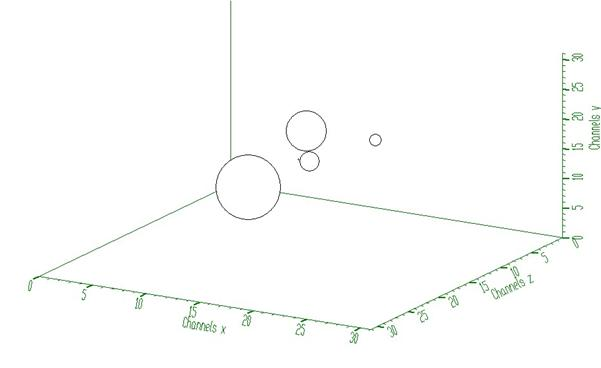Fig. 4 Spectrum from Fig. 2 after boosted deconvolution (10 iterations repeated 10 times). It decomposes completely cluster of peaks from Fig 2.

Script:

// Example to illustrate the Gold deconvolution (class TSpectrum3).

// To execute this example, do

// root > .x Decon3_hr.C

void Decon3_hr() {

Int_t i, j, k;

Int_t nbinsx = 32;

Int_t nbinsy = 32;

Int_t nbinsz = 32;

Int_t xmin  = 0;

Int_t xmax  = nbinsx;

Int_t ymin  = 0;

Int_t ymax  = nbinsy;

Int_t zmin  = 0;

Int_t zmax  = nbinsz;

float *** source = new float **[nbinsx];

float *** resp = new float **[nbinsx];

for(i=0;i<nbinsx;i++){

source[i]=new float* [nbinsy];

for(j=0;j<nbinsy;j++)

source[i][j]=new float [nbinsz];

}

for(i=0;i<nbinsx;i++){

resp[i]=new float* [nbinsy];

for(j=0;j<nbinsy;j++)

resp[i][j]=new float [nbinsz];

}

TH3F *decon_in = new TH3F("decon_in","Deconvolution",nbinsx,xmin,xmax,nbinsy,ymin,ymax,nbinsz,zmin,zmax);

TH3F *decon_resp = new TH3F("decon_resp","Deconvolution",nbinsx,xmin,xmax,nbinsy,ymin,ymax,nbinsz,zmin,zmax);

TFile *f = new TFile("TSpectrum3.root");

decon_in=(TH3F*) f->Get("decon_in;1");

decon_resp=(TH3F*) f->Get("decon_resp;1");

TCanvas *Deconvolution = new TCanvas("Deconvolution","High resolution deconvolution of 3-dimensional spectra",10,10,1000,700);

TSpectrum3 *s = new TSpectrum3();

for (i = 0; i < nbinsx; i++){

for (j = 0; j < nbinsy; j++){

for (k = 0; k < nbinsz; k++){

source[i][j][k] = decon_in->GetBinContent(i + 1,j + 1,k + 1);

resp[i][j][k] = decon_resp->GetBinContent(i + 1,j + 1,k + 1);

}

}

}

s->Deconvolution(source,resp,nbinsx,nbinsy,nbinsz,10,10,2);

for (i = 0; i < nbinsx; i++){

for (j = 0; j < nbinsy; j++){

for (k = 0; k < nbinsz; k++){

decon_in->SetBinContent(i + 1,j + 1,k + 1, source[i][j][k]);

}

}

}

decon_in->Draw("");

}

Int_t SearchHighRes(const float*** source, float*** dest, Int_t ssizex, Int_t ssizey, Int_t ssizez, Double_t sigma, Double_t threshold, Bool_t backgroundRemove, Int_t deconIterations, Bool_t markov, Int_t averWindow)
```THREE-DIMENSIONAL HIGH-RESOLUTION PEAK SEARCH FUNCTION
This function searches for peaks in source spectrum
It is based on deconvolution method. First the background is
removed (if desired), then Markov spectrum is calculated
(if desired), then the response function is generated
according to given sigma and deconvolution is carried out.
It returns number of found peaks.

Function parameters:
source-pointer to the matrix of source spectrum
dest-pointer to the matrix of resulting deconvolved spectrum
ssizex-x length of source spectrum
ssizey-y length of source spectrum
ssizez-z length of source spectrum
sigma-sigma of searched peaks, for details we refer to manual
threshold-threshold value in % for selected peaks, peaks with
amplitude less than threshold*highest_peak/100
are ignored, see manual
backgroundRemove-logical variable, set if the removal of
background before deconvolution is desired
deconIterations-number of iterations in deconvolution operation
markov-logical variable, if it is true, first the source spectrum
is replaced by new spectrum calculated using Markov
chains method.
averWindow-averanging window of searched peaks, for details
we refer to manual (applies only for Markov method)

```

Peaks searching

Goal: to identify automatically the peaks in spectrum with the presence of the continuous background, one- and two-fold coincidences (ridges) and statistical fluctuations - noise.

The common problems connected with correct peak identification in three-dimensional coincidence spectra are

• non-sensitivity to noise, i.e., only statistically relevant peaks should be identified
• non-sensitivity of the algorithm to continuous background
• non-sensitivity to one-fold coincidences (coincidences peak  peak  background in all dimensions) and their crossings
• non-sensitivity to two-fold coincidences (coincidences peak  background  background in all dimensions) and their crossings
• ability to identify peaks close to the edges of the spectrum region
• resolution, decomposition of doublets and multiplets. The algorithm should be able to recognize close positioned peaks.

Function:

Int_t TSpectrum3::SearchHighRes (const float ***fSource,float ***fDest, int fSizex, int fSizey, int fSizez, float fSigma, double fThreshold, bool fBackgroundRemove,int fDeconIterations, bool fMarkov, int fAverWindow)

This function searches for peaks in source spectrum. It is based on deconvolution method. First the background is removed (if desired), then Markov smoothed spectrum is calculated (if desired), then the response function is generated according to given sigma and deconvolution is carried out. On success it returns number of found peaks.

Parameters:

fSource-pointer to the matrix of source spectrum

fDest-resulting spectrum after deconvolution

fSizex, fSizey, fSizez -lengths of the source and destination spectra

fSigma-sigma of searched peaks

fThreshold- threshold value in % for selected peaks, peaks with amplitude less than threshold*highest_peak/100 are ignored

fBackgroundRemove- background_remove-logical variable, true if the removal of background before deconvolution is desired

fDeconIterations-number of iterations in deconvolution operation

fMarkov-logical variable, if it is true, first the source spectrum is replaced by new spectrum calculated using Markov chains method

fAverWindow-width of averaging smoothing window

References:

 M.A. Mariscotti: A method for identification of peaks in the presence of background and its application to spectrum analysis. NIM 50 (1967), 309-320.

  M. Morháč, J. Kliman, V. Matouek, M. Veselský, I. Turzo.:Identification of peaks in multidimensional coincidence gamma-ray spectra. NIM, A443 (2000) 108-125.

 Z.K. Silagadze, A new algorithm for automatic photopeak searches. NIM A 376 (1996), 451.

Example of peak searching method

SearchHighRes function provides users with the possibility to vary the input parameters and with the access to the output deconvolved data in the destination spectrum. Based on the output data one can tune the parameters.

Example 1  script Search3.c: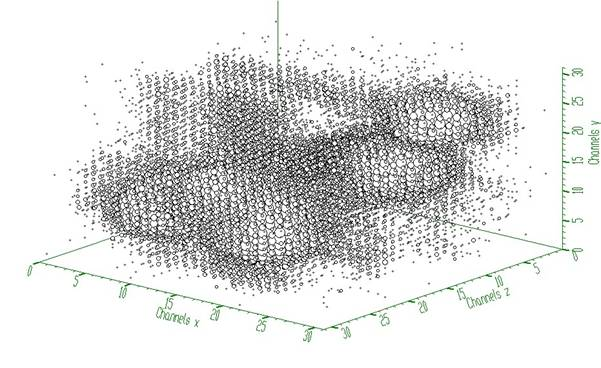Fig. 1 Three-dimensional spectrum with 5 peaks (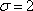, threshold=5%, 3 iterations steps in the deconvolution)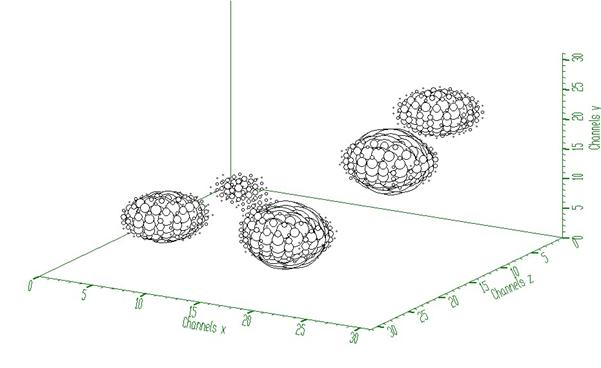Fig. 2 Spectrum from Fig. 1 after background elimination and deconvolution

Script:

// Example to illustrate high resolution peak searching function (class TSpectrum3).

// To execute this example, do

// root > .x Search3.C

void Search3() {

Int_t i, j, k, nfound;

Int_t nbinsx = 32;

Int_t nbinsy = 32;

Int_t nbinsz = 32;

Int_t xmin  = 0;

Int_t xmax  = nbinsx;

Int_t ymin  = 0;

Int_t ymax  = nbinsy;

Int_t zmin  = 0;

Int_t zmax  = nbinsz;

float *** source = new float **[nbinsx];

float *** dest = new float **[nbinsx];

for(i=0;i<nbinsx;i++){

source[i]=new float* [nbinsy];

for(j=0;j<nbinsy;j++)

source[i][j]=new float [nbinsz];

}

for(i=0;i<nbinsx;i++){

dest[i]=new float* [nbinsy];

for(j=0;j<nbinsy;j++)

dest[i][j]=new float [nbinsz];

}

TH3F *search = new TH3F("Search","Peak searching",nbinsx,xmin,xmax,nbinsy,ymin,ymax,nbinsz,zmin,zmax);

TFile *f = new TFile("TSpectrum3.root");

search=(TH3F*) f->Get("search2;1");

TCanvas *Search = new TCanvas("Search","Peak searching",10,10,1000,700);

TSpectrum3 *s = new TSpectrum3();

for (i = 0; i < nbinsx; i++){

for (j = 0; j < nbinsy; j++){

for (k = 0; k < nbinsz; k++){

source[i][j][k] = search->GetBinContent(i + 1,j + 1,k + 1);

}

}

}

nfound = s->SearchHighRes(source, dest, nbinsx, nbinsy, nbinsz, 2, 5, kTRUE, 3, kFALSE, 3);

printf("Found %d candidate peaks\n",nfound);

for (i = 0; i < nbinsx; i++){

for (j = 0; j < nbinsy; j++){

for (k = 0; k < nbinsz; k++){

search->SetBinContent(i + 1,j + 1,k + 1, dest[i][j][k]);

}

}

}

Float_t *PosX = new Float_t[nfound];

Float_t *PosY = new Float_t[nfound];

Float_t *PosZ = new Float_t[nfound];

PosX = s->GetPositionX();

PosY = s->GetPositionY();

PosZ = s->GetPositionZ();

for(i=0;i<nfound;i++)

printf("posx= %d, posy= %d, posz= %d\n",(int)(PosX[i]+0.5), (int)(PosY[i]+0.5), (int)(PosZ[i]+0.5));

search->Draw("");

}

Int_t SearchFast(const float*** source, float*** dest, Int_t ssizex, Int_t ssizey, Int_t ssizez, Double_t sigma, Double_t threshold, Bool_t markov, Int_t averWindow)
TH1 * GetHistogram() const
`{return fHistogram;}`
Int_t GetNPeaks() const
`{return fNPeaks;}`
Float_t * GetPositionX() const
`{return fPositionX;}`
Float_t * GetPositionY() const
`{return fPositionY;}`
Float_t * GetPositionZ() const
`{return fPositionZ;}`